State True or False.Consider the shape below.Different views of the above shape are:      State whether the figures of different views of the given shape are true or false?

# State True or False.Consider the shape below.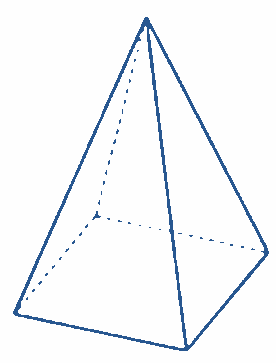Different views of the above shape are: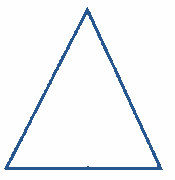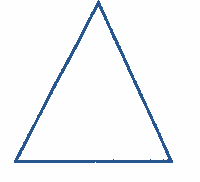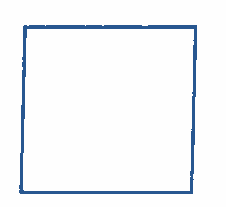State whether the figures of different views of the given shape are true or false?

1. A
True
2. B
False

Fill Out the Form for Expert Academic Guidance!l

+91

Live ClassesBooksTest SeriesSelf Learning

Verify OTP Code (required)

### Solution:

The given diagram is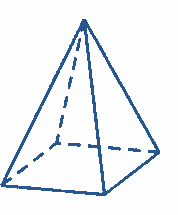We know that 3-D objects have distinct perspectives from different locations, which are referred to as the top view, side view, and front view.
The front view of the given figure isThe side view of the given figure isThe top view of the given figure is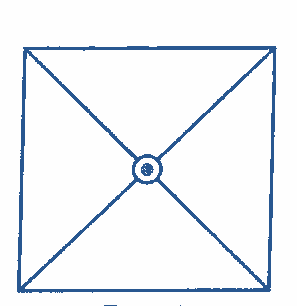Therefore, the given views,are false.
The correct option is (2).

## Related content

 Difference Between Distance and Displacement Difference Between Mass and Weight Differences & Comparisons Articles in Physics Important Topic of Physics: Reynolds Number Distance Speed Time Formula Refractive Index Formula Mass Formula Electric Current Formula Electric Power Formula Resistivity Formula+91

Live ClassesBooksTest SeriesSelf Learning

Verify OTP Code (required)